All isogonal circum-strophoids with a node at I are nK(X6, R, X1) = cK(#X1, R) with their root R on the trilinear polar of the Gergonne point X(7) containing the points X(241), X(514), X(650), X(665), X(905), X(1323), X(1375), X(1465), X(1638), X(3002), X(3004), X(3008), X(3015), etc. See Special Isocubics §4.3.2. See also Table 69 for other cK(#X1, R) which are not necessarily strophoids. For non-isogonal circum-strophoids, see CL038. See also CL001, CL027 and a generalization in the page P-conical cubics.   Each isogonal circum-strophoid is : • the locus of foci of inscribed conics centered on a line (L) passing through the incenter I = X(1) and another point P = (p : q : r). Note that R is the complement of the isotomic conjugate of the trilinear pole of (L). The trilinear polar of R is tangent to the incircle. • spK(Z, X1) where Z is the infinite point of (L), see CL055 and more generally spK(Z, Q) where Q is any point on (L). The singular focus F lies on the circumcircle of ABC since it is the isogonal conjugate of Z. • the locus of common points of a circle passing through X(1) with center Ω on (L) and the line FΩ. • the pedal curve, with respect to X(1), of the parabola (P) with directrix (L) and focus the reflection of X(1) about F. (P) is the pivotal conic of the strophoid and it is inscribed in the excentral triangle. The tangents through X(1) to (P) are the nodal tangents of the strophoid. • the inverse in the incircle of the rectangular hyperbola passing through X(1) with tangent (L), and the inverses of A, B, C in the incircle.   These strophoids form a pencil of focal cubics whose equation is : (a^2 y z + b^2 z x + c^2 x y) ∑ p (c y - b z) = (x + y + z) ∑ b c p x (c y - b z), where ∑ p (c y - b z) = 0 is the equation of the line passing through I and P, and ∑ b c p x (c y - b z) = 0 is the equation of the circumconic which is the isogonal conjugate of the previous line i.e. the circumconic passing through I and P*. The following table shows a selection of such remarkable strophoids.P root R focus F infinity Z cubic or other centers on the cubic remarks X(2) X(514) X(106) X(519) K086 Gergonne strophoid nodal tangents parallel to the asymptotes of the Feuerbach hyperbola X(3) X(905) X(104) X(517) X(1339) X(4) X(14837) X(102) X(515) X(2077) X(5) X(10015) X(953) X(952) K165 NPC strophoid X(6) X(650) X(105) X(518) K040 Pelletier strophoid the only nK0 of the pencil nodal tangents parallel to the asymptotes of the rectangular circum-hyperbola with center X(116) X(7) X(7658) X(103) X(516) X(21) X(14838) X(759) X(758) X(484), X(3065) nodal tangents parallel to the asymptotes of the Jerabek hyperbola X(30) X(41800) X(74) X(30) X(39) X(665) X(14665) X(14839) K359 Brocard strophoid X(88) X(3960) X(2718) X(2802) X(2718) X(142) X(3676) X(1477) X(5853) X(2078) X(190) X(4763) X(2382) X(537) X(537) X(474) X(3669) X(8686) X(3880) X(1319), X(1320) X(521) X(1465) X(108) X(521) X(522) X(3911) X(109) X(522) X(525) X(1375) X(112) X(525) X(528) X(1638) X(840) X(528) X(7), X(55) X(740) X(4369) X(741) X(740) nodal tangents parallel to the asymptotes of the Kiepert hyperbola X(905) X(241) X(934) X(3900) X(918) X(26007) X(919) X(918) X(2284)Related lociLet S(Z) be the isogonal strophoid with infinite point Z, focus F = Z* on the circumcircle (O). Its orthic line L passes through X(1), Z and its real asymptote A(Z) is the homothetic of L under h(F, 2). S(Z) meets A(Z) again at X whose isogonal conjugate Y = X* is the intersection of the parallel at F to L and the perpendicular at X(1) to L. The loci below are obtained when Z traverses the line at infinity.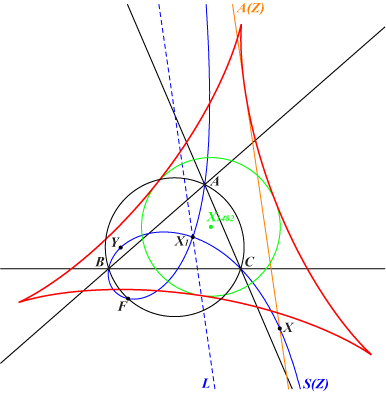Envelope of the real asymptote A(Z) envelopes a deltoid which is the reflection about X(1) of the anticomplement aH3 of the Steiner deltoid H3. The circle with center X(1482), radius R is the incircle of the deltoid. Note that aH3 is the envelope of the line FY.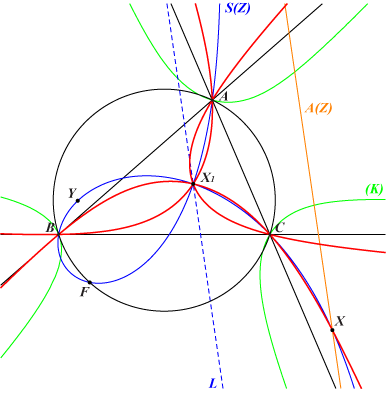Locus of X S(Z) meets A(Z) at X which lies on a circular circum-quintic (Q) passing through the triple point X(1). The tangents at X(1) are perpendicular to the internal bisectors of ABC. A, B, C are double points. (Q) meets the line at infinity again at three points which lie on a cubic (K) = nK0(X6, X1 x X2550, ?). (Q) meeets the sidelines of ABC again at three collinear points lying on the trilinear polar of X(8056).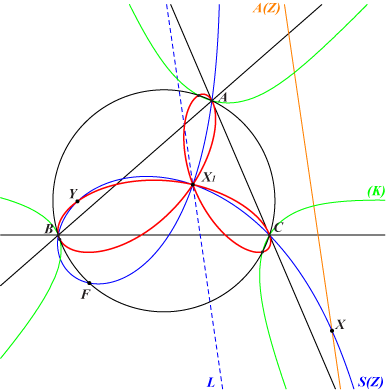Locus of Y The isogonal conjugate Y of X lies on a trifolium (T) with triple point at X(1) which is obviously the isogonal transform of the quintic above. The nodal tangents are perpendicular to the internal bisectors of ABC. (T) is the pedal curve of aH3 with respect to X(1) i.e. the locus of the foot of a perpendicular dropped from X(1) to the tangents to aH3. (T) is a bicircular circum-quartic meeting the circumcircle (O) at the same points as the cubic (K) above. (T) meets the sidelines of ABC again at six points lying on a same (rather uninteresting) conic.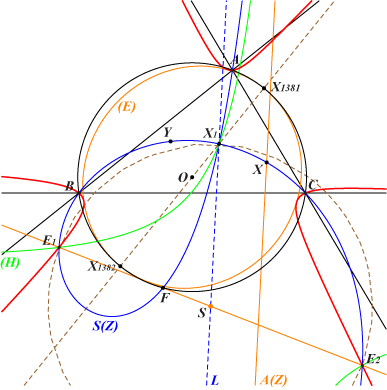Centers of anallagmaty The real centers of anallagmaty E1, E2 lie on the cubic K407. They obviously also lie on the rectangular hyperbola (H) which is the polar conic of Z in S(Z). They are the common points of the perpendicular at F to X(1)F (meeting L at S) and the circle with center S passing through S. This perpendicular envelopes the ellipse (E) which is the pivotal conic of K407. (E) is the MacBeath ellipse of the excentral triangle. E1, E2 are isogonal conjugate points on S(Z).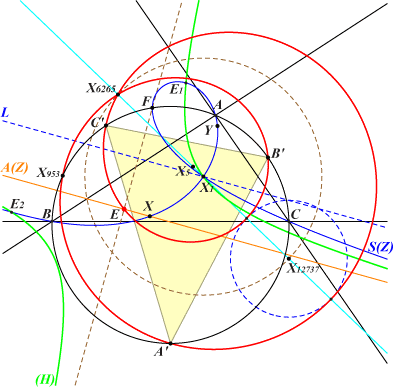Center E of (H) The center E of the polar conic (H) of Z in S(Z) lies on the real asymptote A(Z). It is the reflection of F about L. The locus (E) of E is a limaçon of Pascal with node X(6265) obtained when Z is an infinite point of the circum-conic passing through X(100) and X(1389). (E) meets the circumcircle again at X(953) and the vertices A', B', C' of the circumcevian triangle of X(1). It axis of symmetry is the line X(1)X(5)X(6265) and the two remaining common points lie on the (dashed blue) circle with center X(12737) passing through X(104), X(106), X(1320), etc.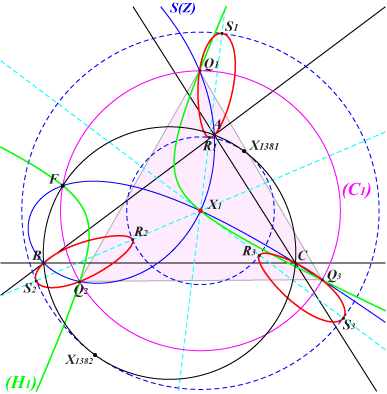Equilateral triangles inscribed in S(Z) Let (C1) be the circle with center X(1) passing through F. The inverse of S(Z) in the circle (C1) is the rectangular hyperbola (H1) passing through F and meeting S(Z) and (C1) again at the vertices Q1, Q2, Q3 of an equilateral triangle. The locus of Q1, Q2, Q3 is a bicircular circum-quartic (Q) with an isolated singular point X(1). (Q) is tritangent to the two circles with center X(1) passing through X(1381), X(1382). The contacts Ri, Si (i = 1, 2, 3) lie on three parallels at X(1) to the asymptotes of the McCay cubic K003. These parallels are three axes of symmetry of (Q) which is invariant under the rotations with center X(1), and with angles +/- 2π/3. (Q) meets the circumcircle of ABC at the same points as the cubic (K) we met above. *** The isogonal conjugates Q1*, Q2*, Q3* of Q1, Q2, Q3 are obviously three other points on S(Z). They lie on the perpendicular bisector (D) of X(1), F and on the conic (H2) which is its isogonal transform. The locus of Q1*, Q2*, Q3* is the isogonal transform (Q*) of (Q) above. (Q*) is a circular circum-quintic passing through • the infinite points of (K), • X(1) which is an isolated point, • the traces A', B', C' of the trilinear polar of X(88).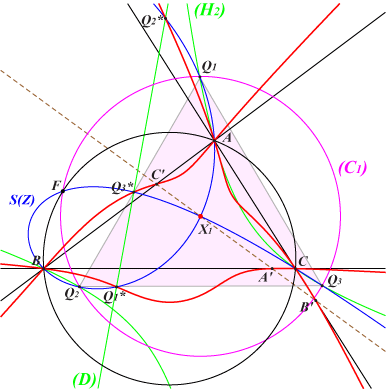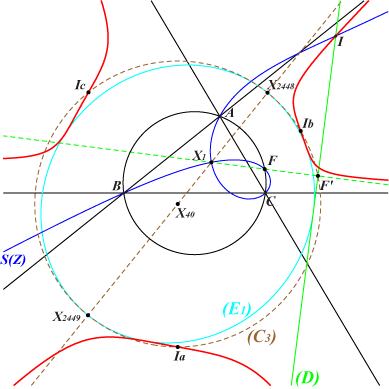Points of inflexion of S(Z) Since S(Z) is a crunodal cubic, it has only one real point of inflexion I. I lies on the perpendicular (D) to X(1)F at the reflection F' of X(1) about F. The envelope of (D) is the ellipse (E1), the homothetic of the MacBeath ellipse under h(X1, 2). The locus of I is a circular quintic meeting the line at infinity again at the same points as the cubic (K) above. X(1) is an isolated double point on the curve. (C3) is the excentral circle with center X(40) and radius 2R.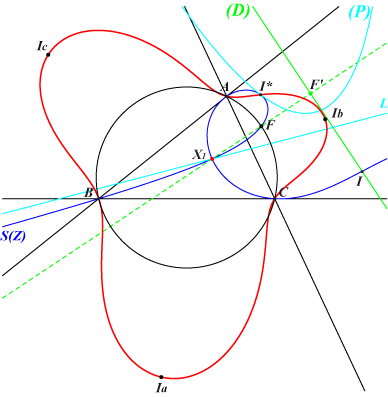The pivotal conic of S(Z) is the parabola (P) with focus F' and directrix the line L passing through Z and F. This parabola is inscribed in the excentral triangle IaIbIc. The isogonal conjugate I* of the real point of inflexion I is the contact of (P) and S(Z) hence the line II* is tangent to (P) and S(Z). The locus of I* is a bicircular circum-quartic meeting the circumcircle again at the same points as the cubic (K) above. It is obviously the isogonal transform of the quintic above. X(1) is an isolated double point on the curve.